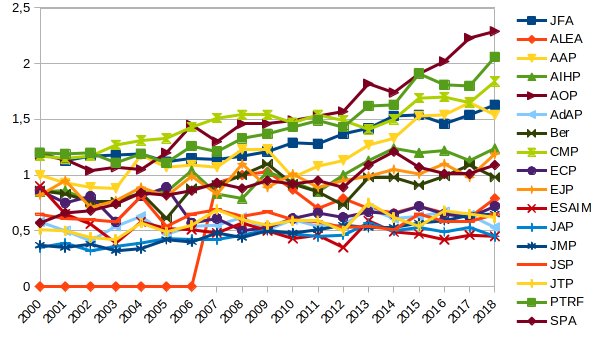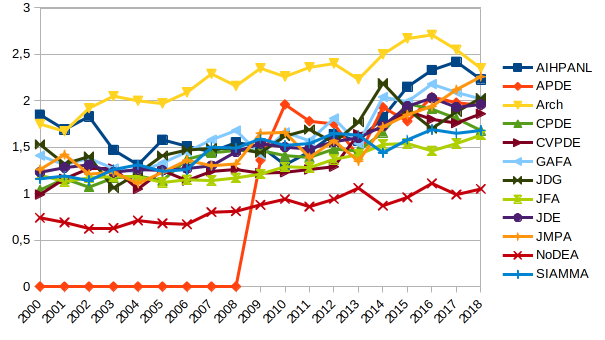# Month: December 2019

This post gives below the Mathematical Citation Quotient (MCQ) from 2000 to 2018 for journals in probability, statistics, analysis, and general mathematics. The numbers were obtained using home brewed scripts and MathSciNet data. The graphics were created with LibreOffice.

Recall that the MCQ is a ratio of two counts for a selected journal and a selected year.  The MCQ for year $Y$ and journal $J$ is given by the formula $\mathrm{MCQ}=m/n$ where

• $m$ is the total number of citations of papers published in jounal $J$ in years $Y-1$,…,$Y-5$ by papers published in year $Y$ in any journal known by MathSciNet;
• $n$ is the total number of papers published in journal $J$ in years $Y-1$,…,$Y-5$.

The Mathematical Reviews compute every year the MCQ for every indexed journal, and make it available on MathSciNet. This formula is very similar to the one of the five years impact factor, the main difference being the population of journals which is specifically mathematical for the MCQ (reference list journals) and the way the citations are extracted. Both biases are negative.

The MCQ is a rough measurement of the social scientific value of journals. The results are quite compatible with what we have in mind. The trends are sometimes intriguing. For probability journals, for instance, it seems that there are three groups. This reminds reinforcement or self-organized criticality. The first group is AOP-PTRF-CMP-JFA-AAP, with some hesitations and an “Annals” naming effect. The second group is AIHP-EJP-SPA-Bernoulli, the third group is ALEA-ECP-AdAP-JAP-JTP-ESAIM. We observe some transitions from one group to another, for instance, since 2010, AAP moved to the first group while ALEA moved to the third group. The case of ECP is very special since its papers are half the standard size. The MCQ probably underestimates the social value of ECP by a rough factor 2, which is logical if we compare with EJP.

Yes, there are more robust ways to measure the social value of a journal, such as for instance the (recursive) eigenfactor, and it could be interesting to check if the three groups are stable!Σ

Let $X=(X_1,\ldots,X_n)$ be a random vector of $(\mathbb{R}^d)^n$ with density proportional to $$(x_1,\ldots,x_n)\in(\mathbb{R}^d)^n\mapsto\mathrm{e}^{-\beta\sum_{i=1}^nV(x_i)}\prod_{i<j}W(x_i-x_j),$$ where $V,W:\mathbb{R}^d\to\mathbb{R}$ are homogeneous functions, with $W\geq0$. This means that there exist $a,b\geq0$ such that for all $\lambda\geq0$ and $x\in\mathbb{R}^d$, $V(\lambda x)=\lambda^a V(x)$ and $W(\lambda x)=\lambda^bW(x)$. Now, for all $\theta>0$, by the change of variable $x_i=\sqrt[a]{\beta/(\theta+\beta)}y_i$,
\begin{multline*}
\int_{(\mathbb{R}^d)^n}\mathrm{e}^{-(\theta+\beta)\sum_iV(x_i)}\prod_{i<j}W(x_i-x_j)\mathrm{d}x\\
=\Bigr(\frac{\beta}{\theta+\beta}\Bigr)^{\frac{nd}{a}+\frac{n(n-1)a}{2b}}
\int_{(\mathbb{R}^d)^n}\mathrm{e}^{-\beta\sum_iV(y_i)}\prod_{i<j}W(y_i-y_j)\mathrm{d}y.
\end{multline*}
We recognize the Laplace transform of a Gamma distribution, since
$\int_0^\infty\mathrm{e}^{-\theta u}u^{\alpha-1}\mathrm{e}^{-\beta u}\mathrm{d}u =\int_0^\infty u^{\alpha-1}\mathrm{e}^{-(\theta+\beta)u}\mathrm{d}u =\Bigr(\frac{\beta}{\theta+\beta}\Bigr)^\alpha\frac{\Gamma(\alpha)}{\beta^\alpha},$and we obtain
$\sum_iV(X_i)\sim\mathrm{Gamma}\Bigr(\frac{nd}{a}+\frac{n(n-1)b}{2a},\beta\Bigr).$
A remarkable general fact! The case $V=\frac{1}{2}\left|\cdot\right|^2$ and $W=\left|\cdot\right|^\beta$ corresponds to the beta Ginibre gas of random matrix theory. The case $V=\frac{n+1}{2}\log(1+\left|\cdot\right|^2)$ and $W=\left|\cdot\right|^2$ corresponds to the Forrester–Krishnapur spherical gas of random matrix theory.

We could generalize even more,  and replace $(x_1,\ldots,x_n)\mapsto\sum_iV(x_i)$ by a homogenenous $(x_1,\ldots,x_n)\mapsto V(x_1,\ldots,x_n)$ and $(x_1,\ldots,x_n)\mapsto\prod_{i<j}W(x_i-x_j)$ by a homogeneous $(x_1,\ldots,x_n)\mapsto W(x_1,\ldots,x_n)$, in the sense that for some $a,b\geq0$ and all $\lambda\geq0$, $x\in(\mathbb{R}^d)^n$, $V(\lambda x)=\lambda^aV(x)$ and $W(\lambda x)=\lambda^bW(x)$. In this case $X=(X_1,\ldots,X_n)$ has density proportional to $x\in(\mathbb{R}^d)^n\mapsto\mathrm{e}^{-\beta V(x)}W(x)$. This would hide the structure of exchangeable gas with pair-interaction that we had in mind for the examples. But this would give $$V(X)=V(X_1,\ldots,X_n)\sim\mathrm{Gamma}\Bigr((n+b)\frac{d}{a},\beta\Bigr).$$

Epilogue. My first successful attempt to compute the law of $\sum_i V(x_i)$ for Coulomb gases was by using a Langevin dynamics in the beta Ginibre case, in relation with a Cox-Ingersoll-Ross process, an observation that goes back to my work in collaboration with François Bolley and Joaquín Fontbona. I included this computation in a talk at Oberwolfach, and mentioned that I do not have any other proof. After my talk, during the break, Bálint Virág looked at me and said Are you sure that there is no other proof? This remark excited my mind and I realized after few hours of intense thinking that there is a direct proof without dynamics and that it is a fairly general fact for gases, far beyond Coulomb gases. The key point is a normalizing constant trick for probability distributions, learnt twenty years ago from Gérard Letac. This is a typical story in the life of a mathematician.

Last Updated on 2021-12-17

Syntax · Style · .